# RD Sharma Solutions for Class 11 Chapter 5 - Trigonometric Functions Exercise 5.1

In Exercise 5.1 of Chapter 5, we shall discuss problems based on trigonometric functions of a real number, values of trigonometric functions and trigonometric identities. Students who consider Maths to be a difficult subject, analyzing the right method to solve the problem is the best method to secure high marks. The RD Sharma Class 11 Solutions are formulated by our expert tutors at BYJU’S in a step by step format to help students understand the concepts and solve problems in a clear manner. Students can download the PDFs of this chapter for free from the links given below.

## Download the Pdf of RD Sharma Solutions for Class 11 Maths Exercise 5.1 Chapter 5 – Trigonometric Functions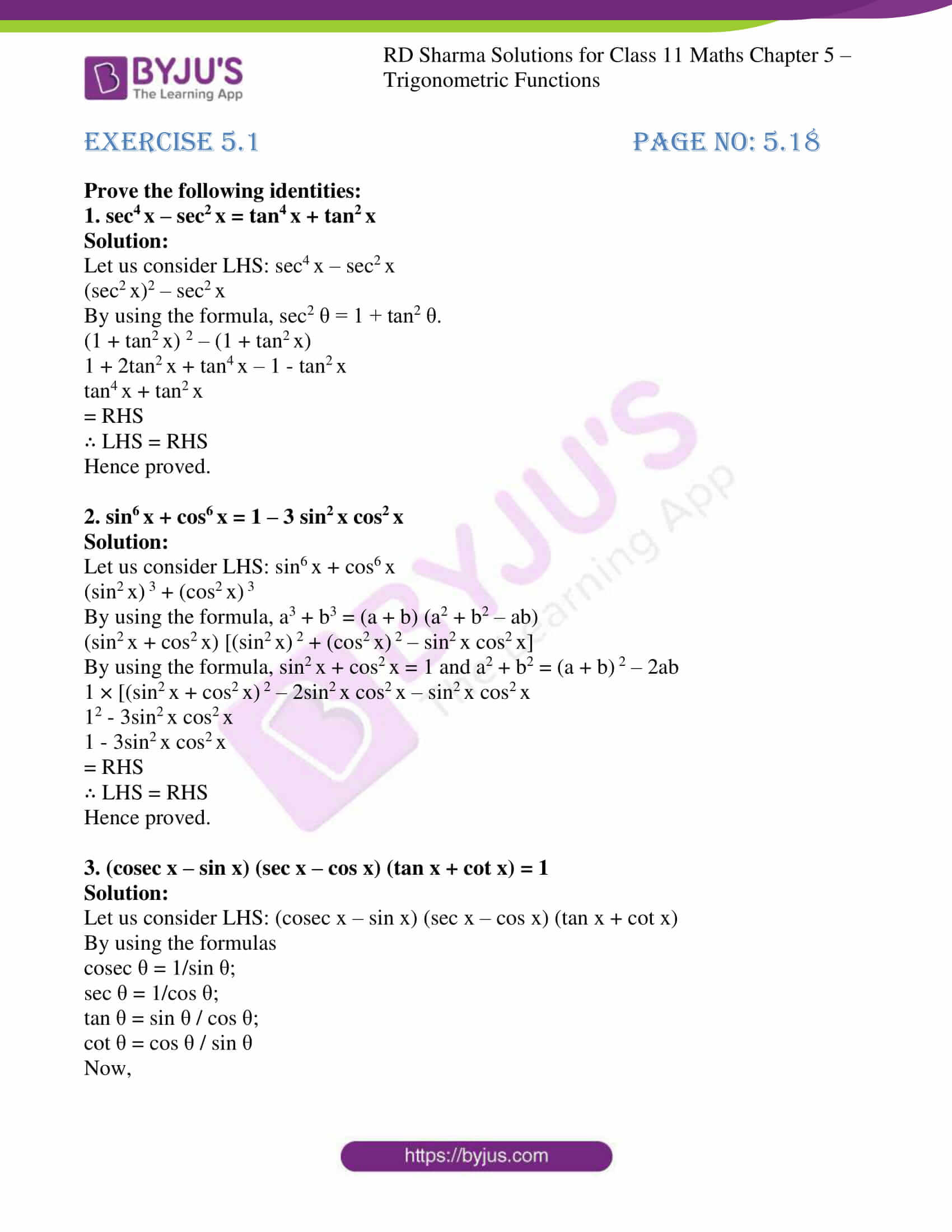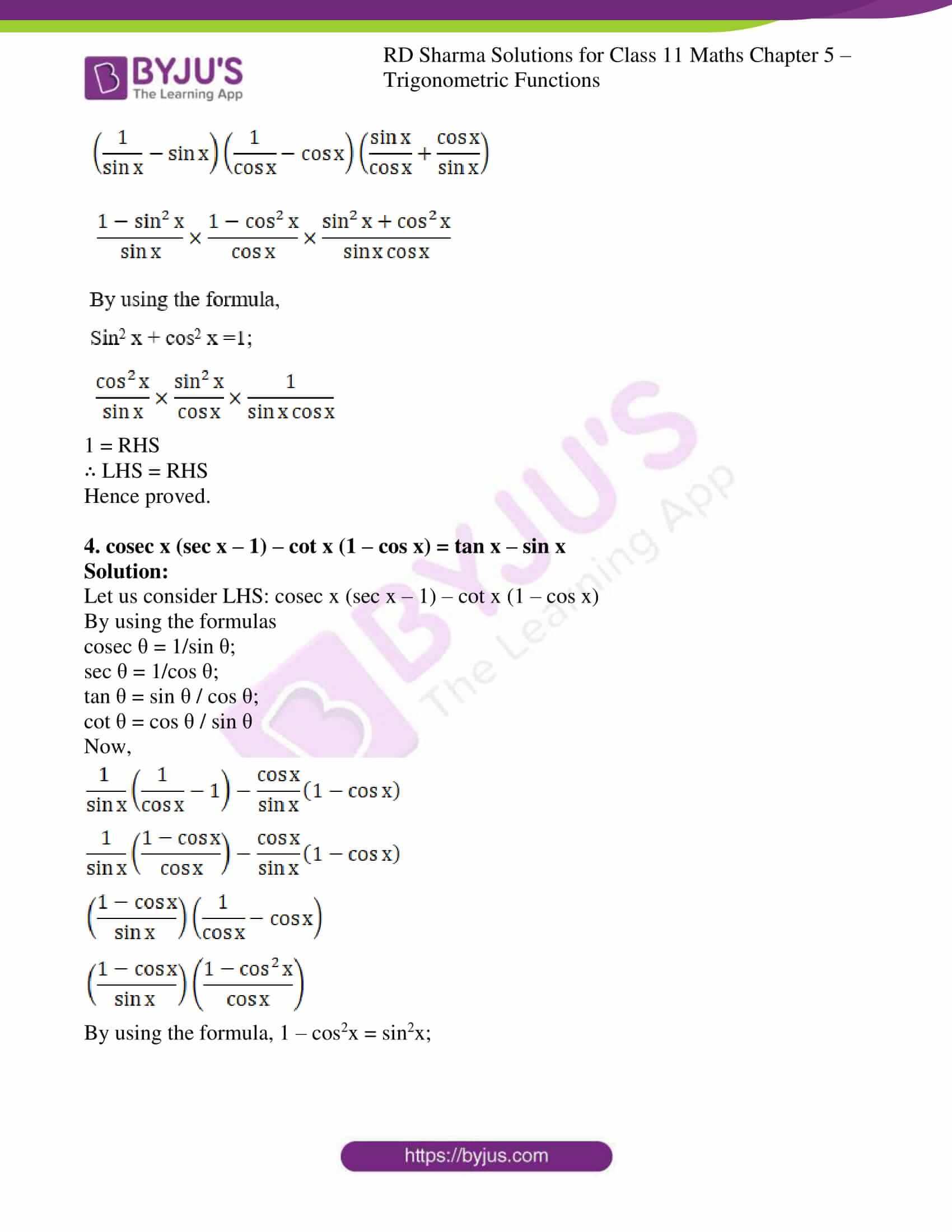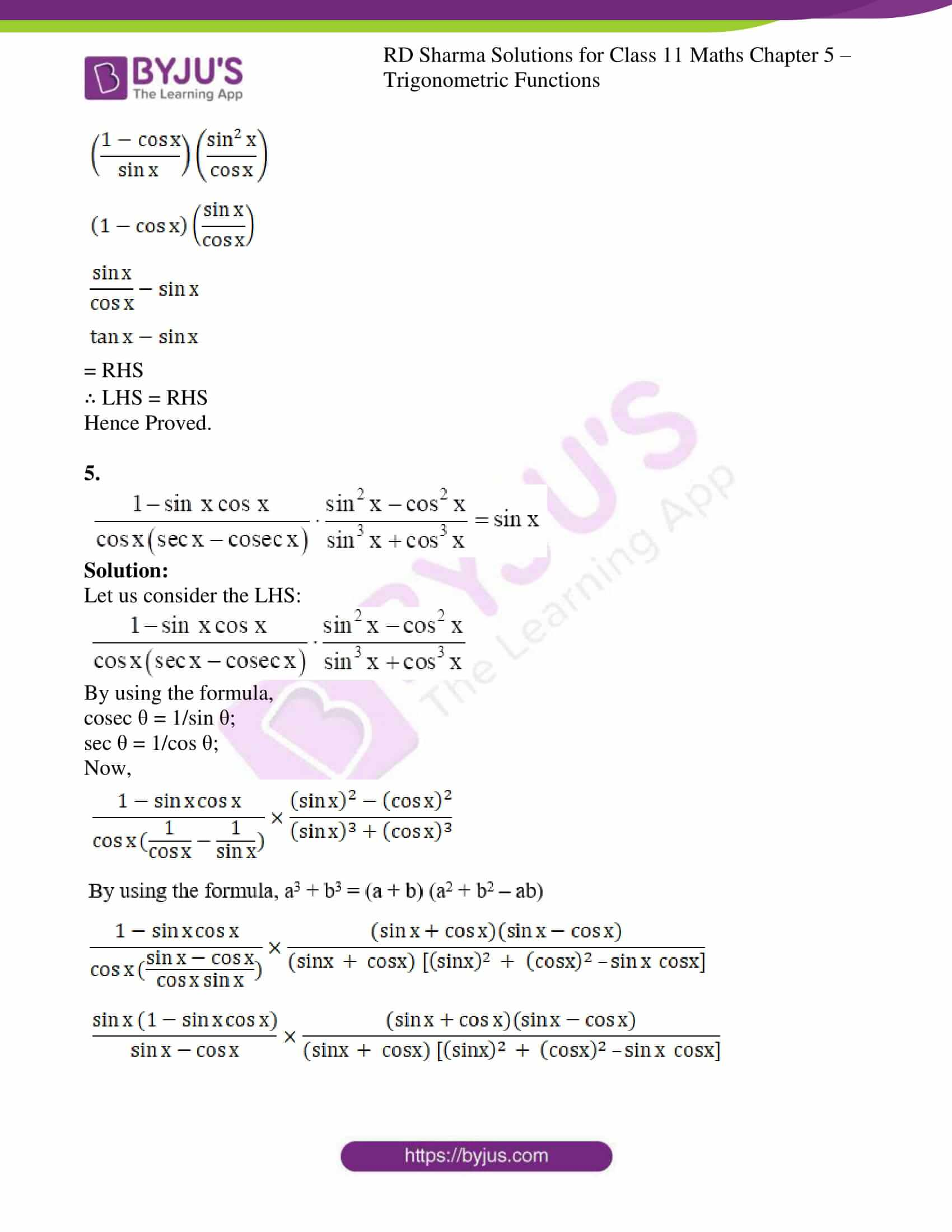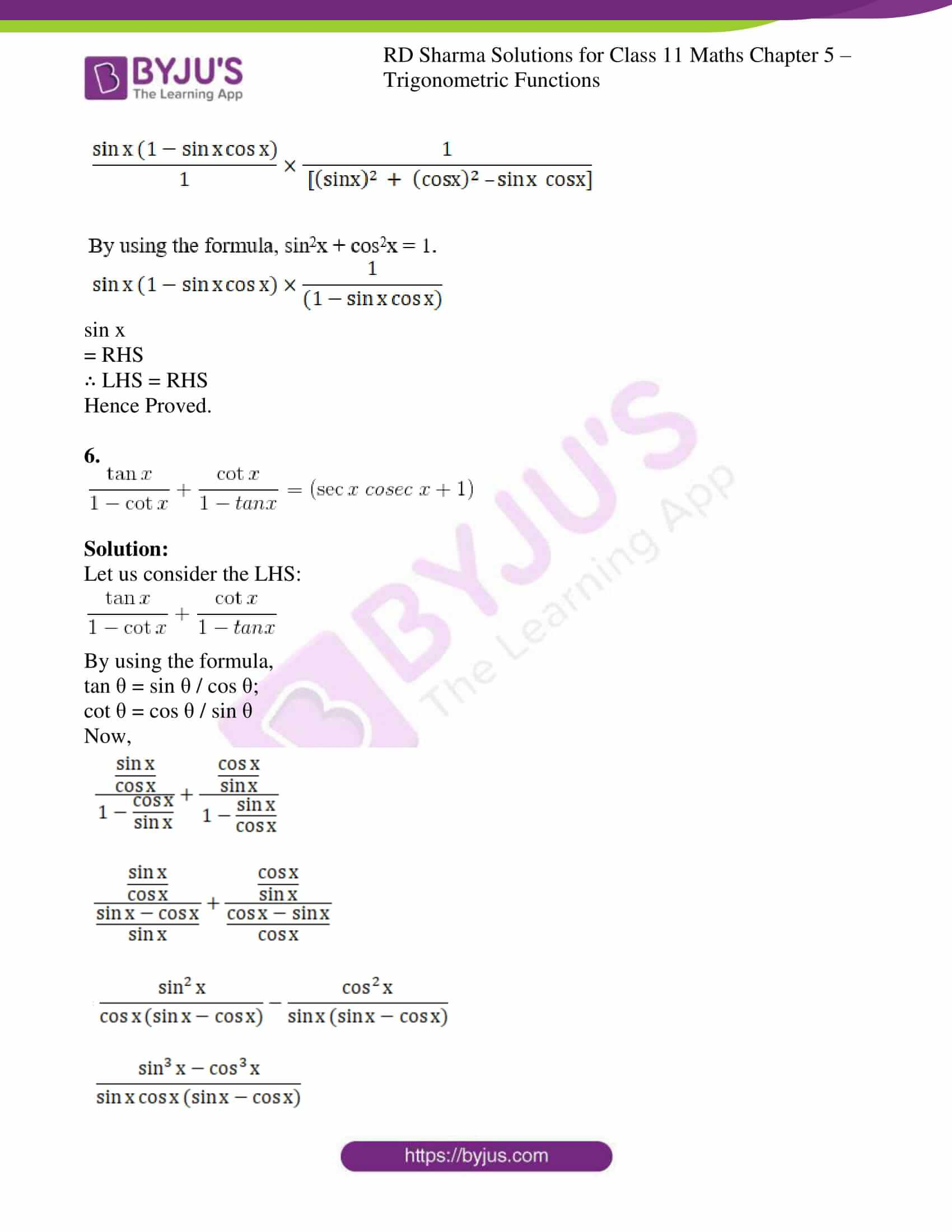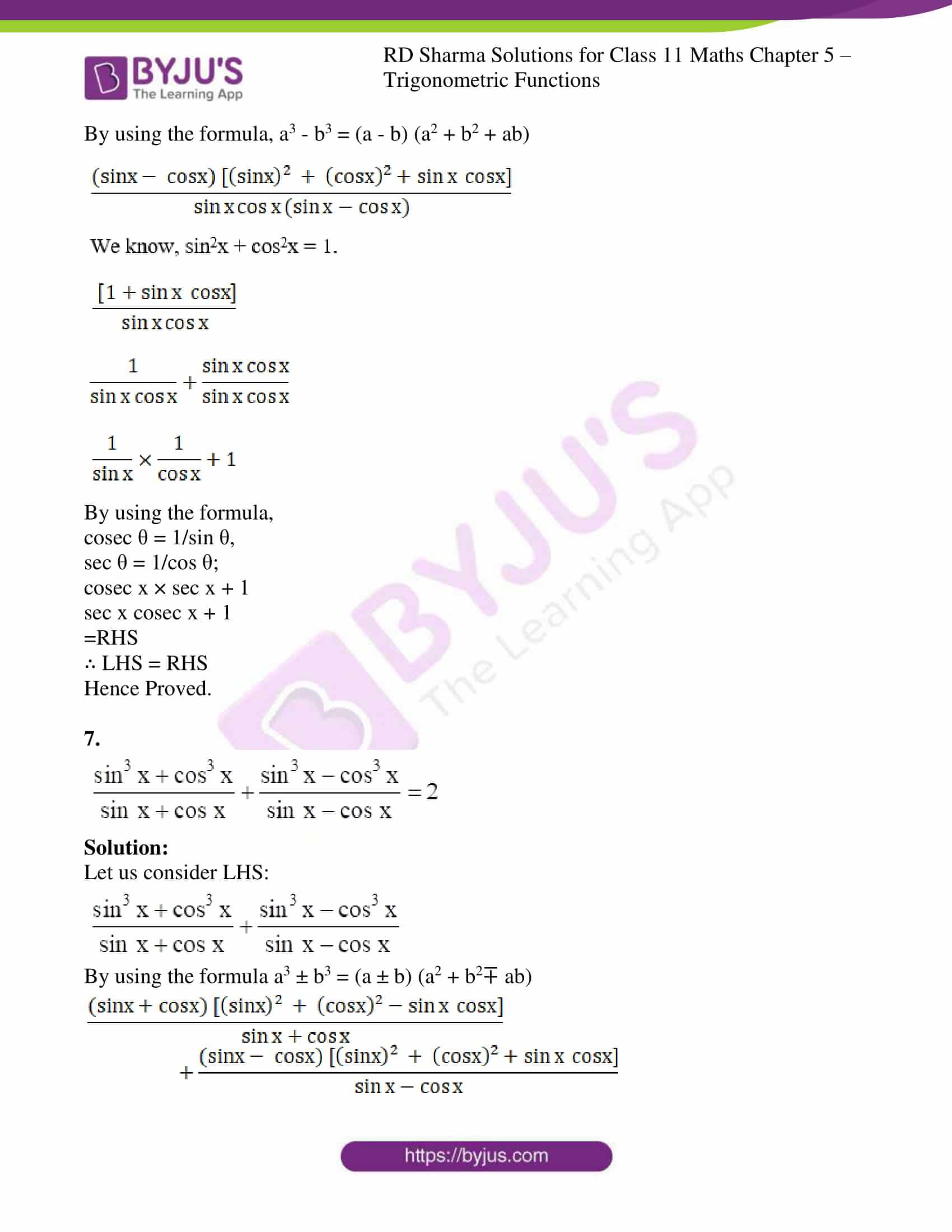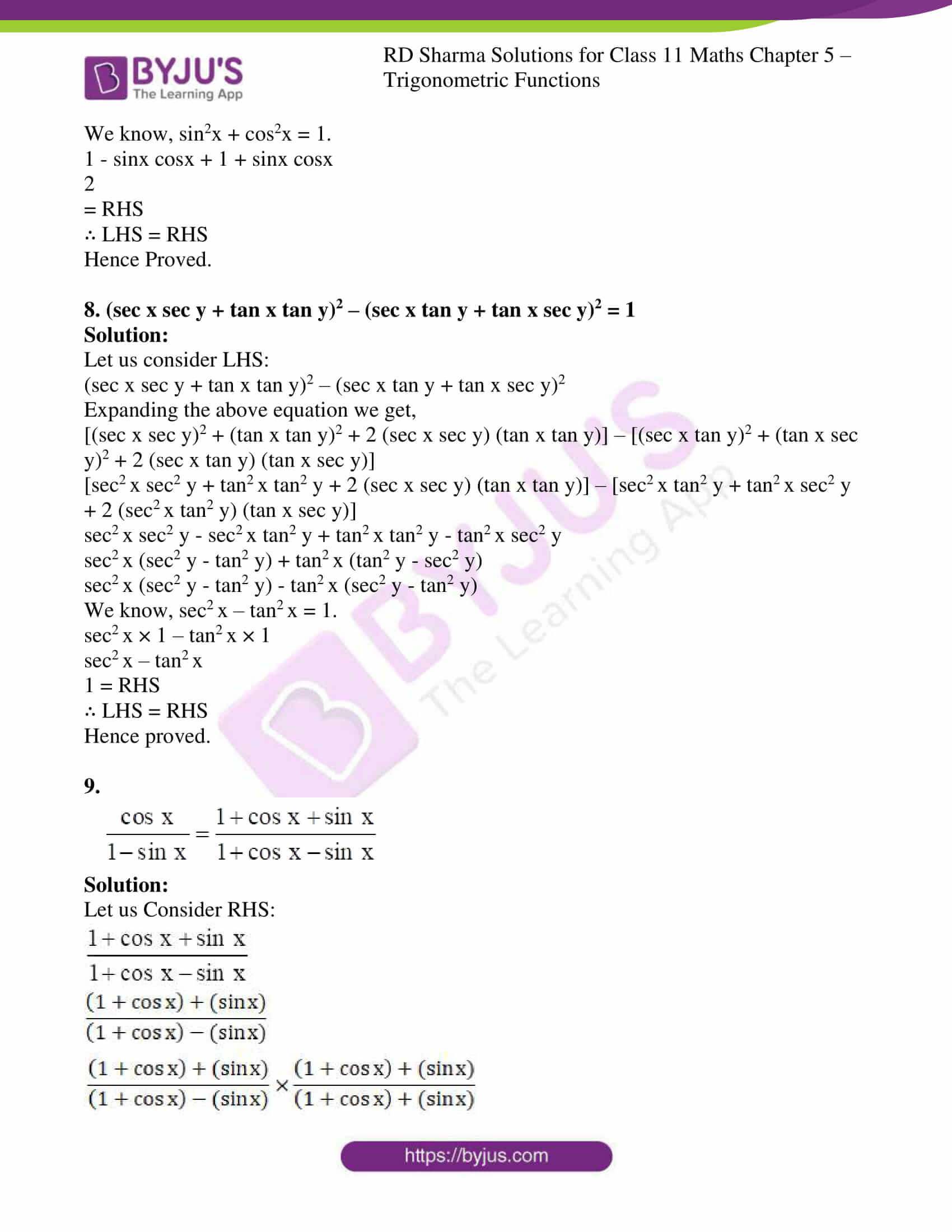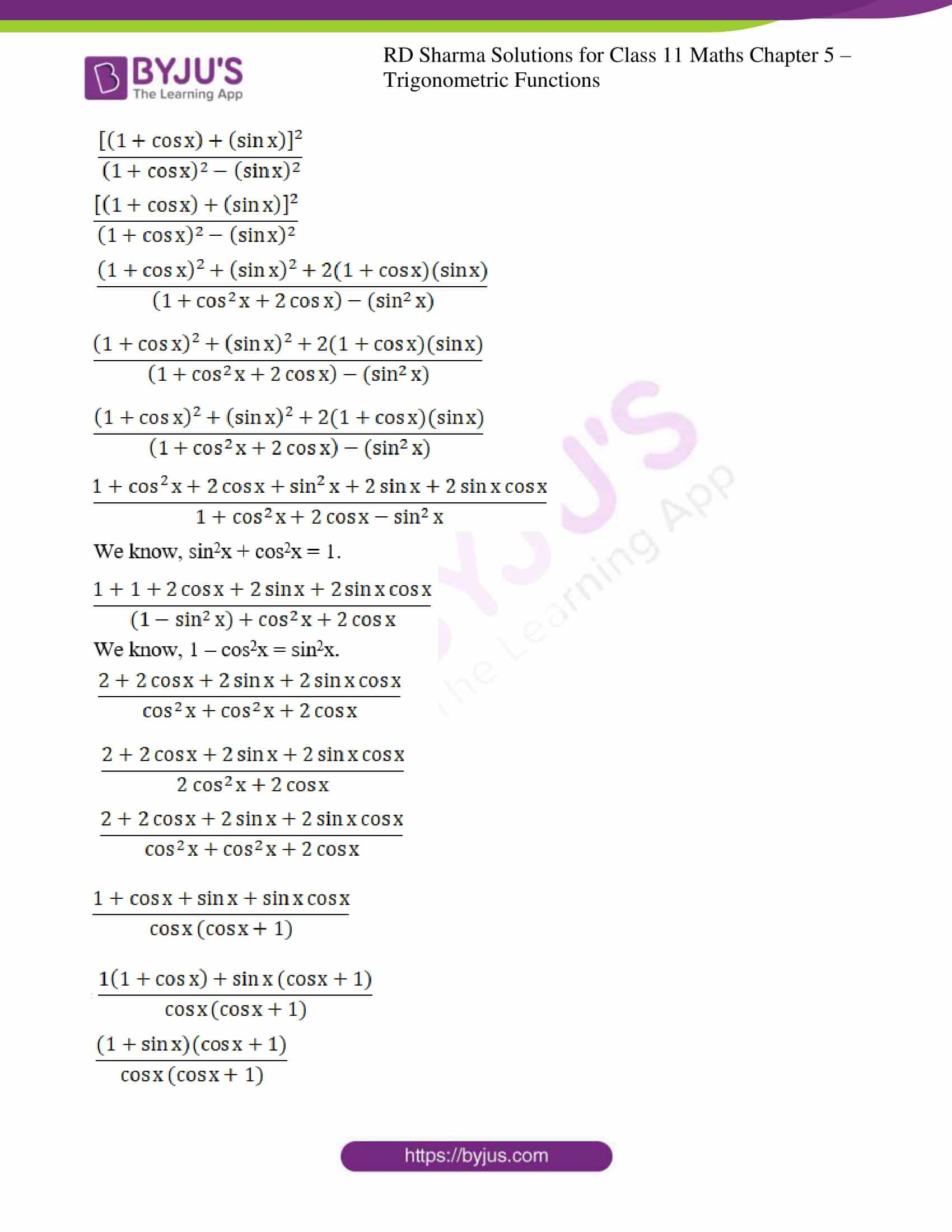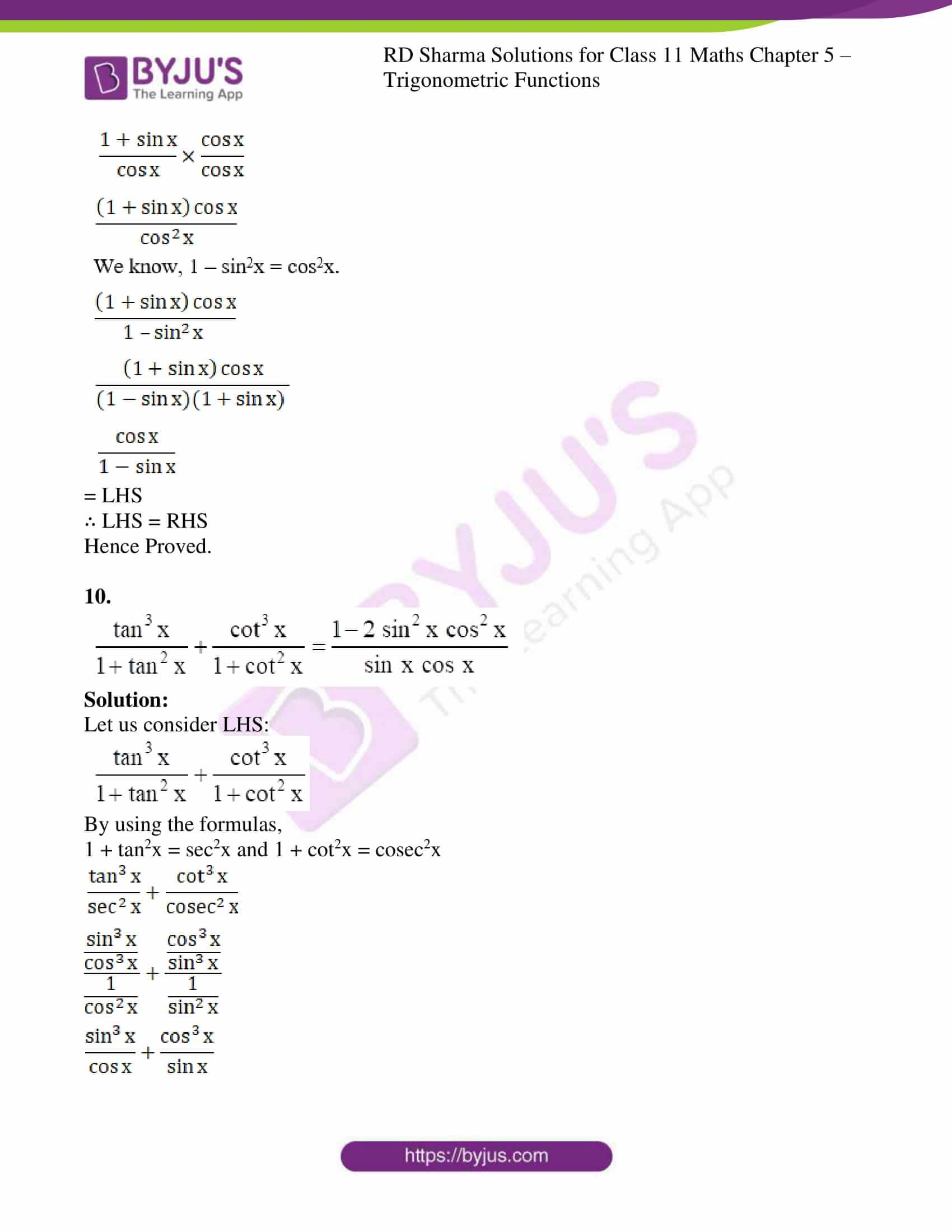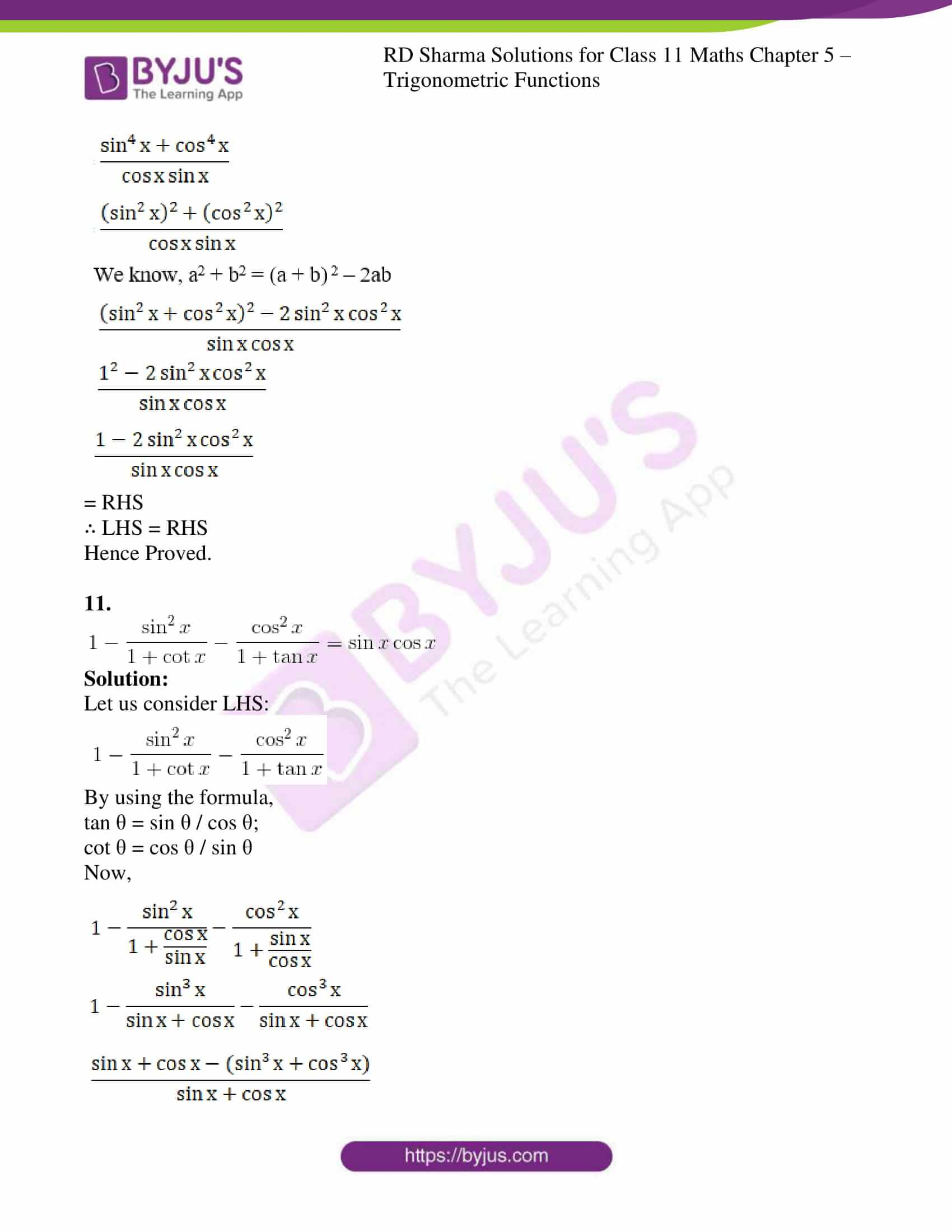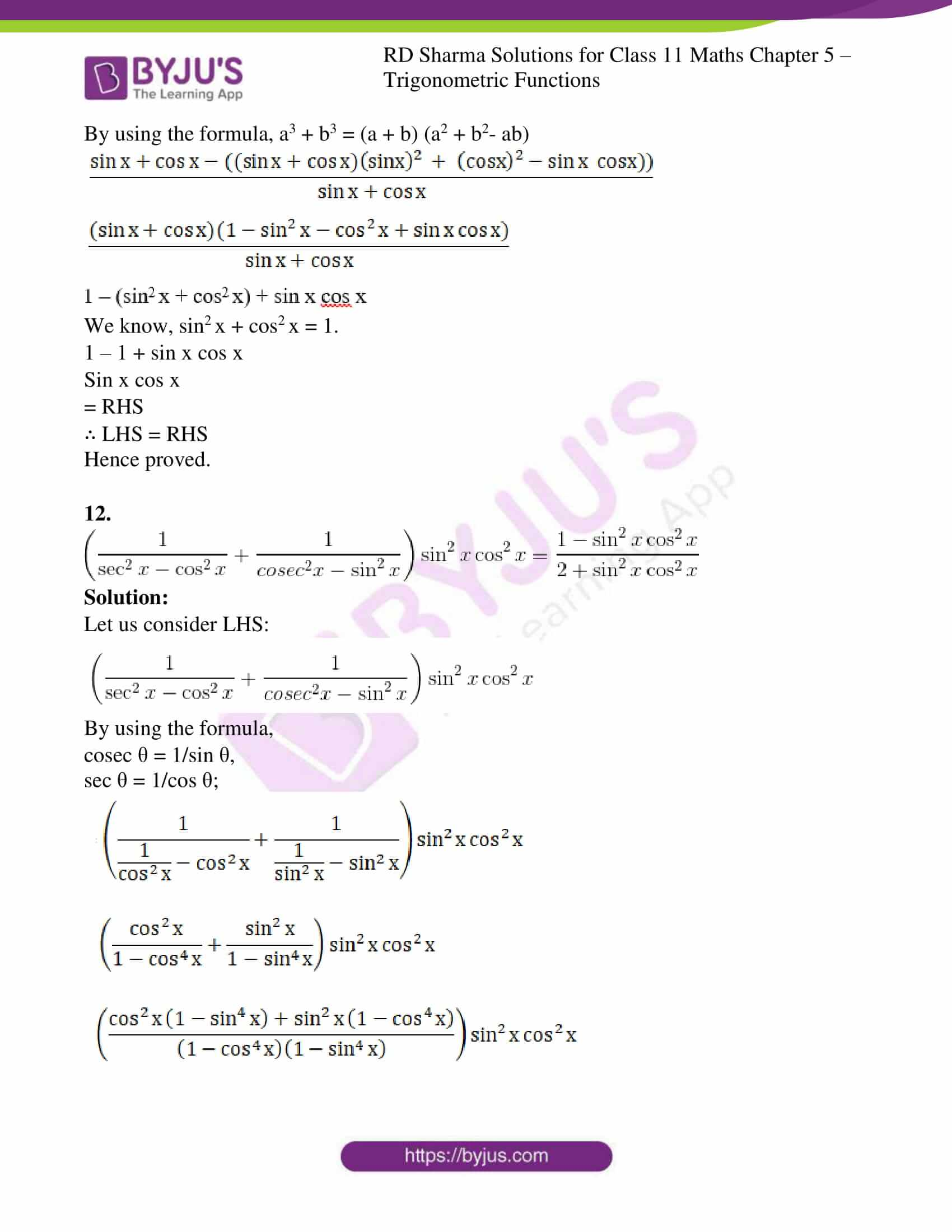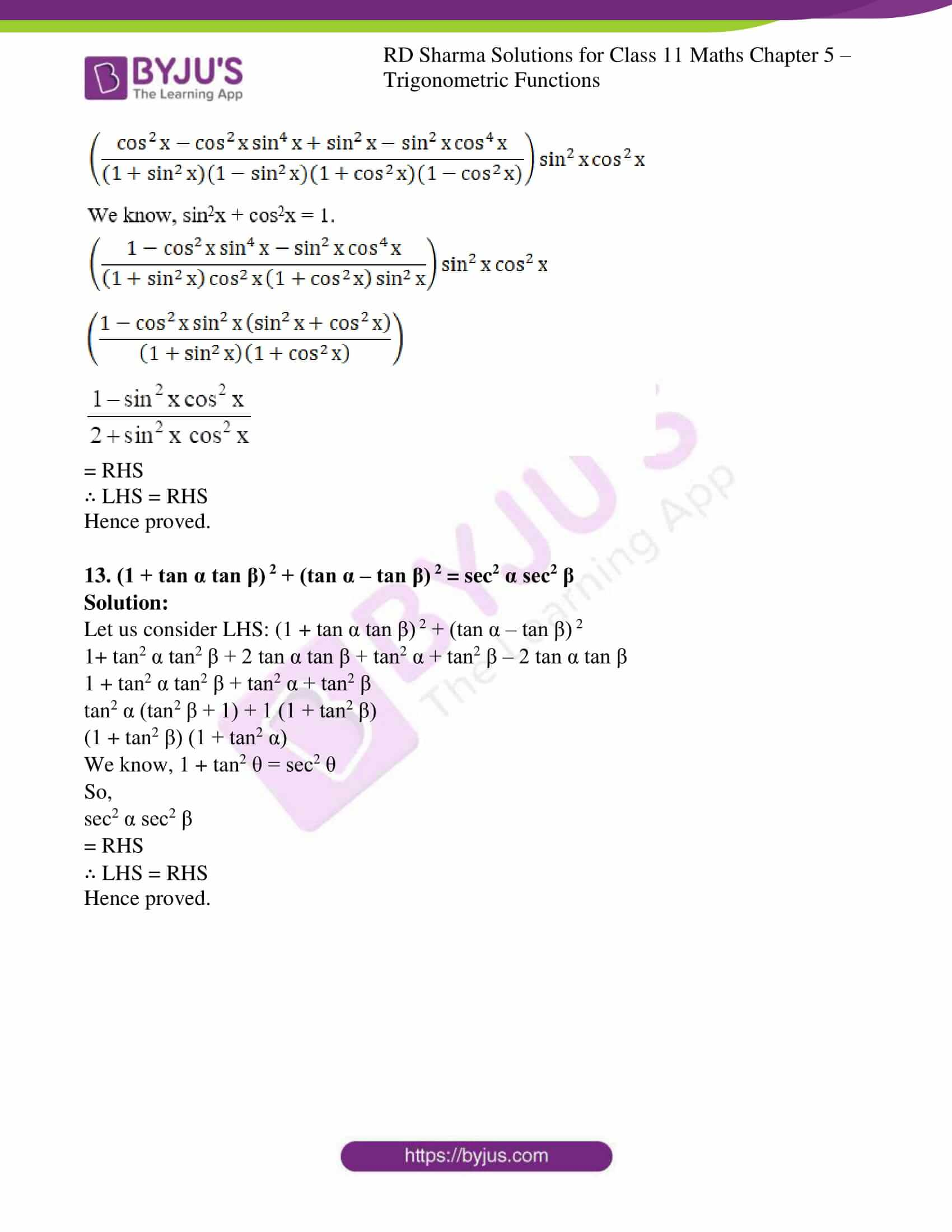### Access answers to RD Sharma Solutions for Class 11 Maths Exercise 5.1 Chapter 5 – Trigonometric Functions

Prove the following identities:

1. sec4 x – sec2 x = tan4 x + tan2 x

Solution:

Let us consider LHS: sec4 x – sec2 x

(sec2 x)2 – sec2 x

By using the formula, sec2 θ = 1 + tan2 θ.

(1 + tan2 x) 2 – (1 + tan2 x)

1 + 2tan2 x + tan4 x – 1 – tan2 x

tan4 x + tan2 x

= RHS

∴ LHS = RHS

Hence proved.

2. sin6 x + cos6 x = 1 – 3 sin2 x cos2 x

Solution:

Let us consider LHS: sin6 x + cos6 x

(sin2 x) 3 + (cos2 x) 3

By using the formula, a3 + b3 = (a + b) (a2 + b2 – ab)

(sin2 x + cos2 x) [(sin2 x) 2 + (cos2 x) 2 – sin2 x cos2 x]

By using the formula, sin2 x + cos2 x = 1 and a2 + b2 = (a + b) 2 – 2ab

1 × [(sin2 x + cos2 x) 2 – 2sin2 x cos2 x – sin2 x cos2 x

12 – 3sin2 x cos2 x

1 – 3sin2 x cos2 x

= RHS

∴ LHS = RHS

Hence proved.

3. (cosec x – sin x) (sec x – cos x) (tan x + cot x) = 1

Solution:

Let us consider LHS: (cosec x – sin x) (sec x – cos x) (tan x + cot x)

By using the formulas

cosec θ = 1/sin θ;

sec θ = 1/cos θ;

tan θ = sin θ / cos θ;

cot θ = cos θ / sin θ

Now,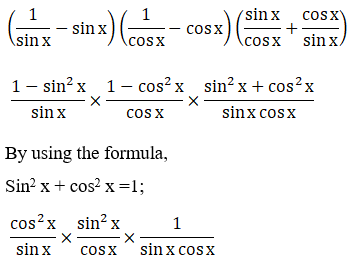1 = RHS

∴ LHS = RHS

Hence proved.

4. cosec x (sec x – 1) – cot x (1 – cos x) = tan x – sin x

Solution:

Let us consider LHS: cosec x (sec x – 1) – cot x (1 – cos x)

By using the formulas

cosec θ = 1/sin θ;

sec θ = 1/cos θ;

tan θ = sin θ / cos θ;

cot θ = cos θ / sin θ

Now,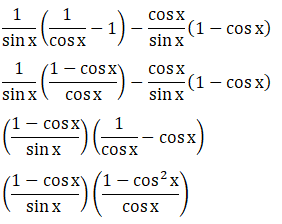By using the formula, 1 – cos2x = sin2x;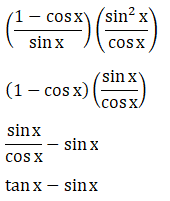= RHS

∴ LHS = RHS

Hence Proved.

5.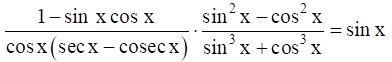Solution:

Let us consider the LHS: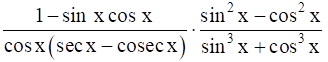By using the formula,

cosec θ = 1/sin θ;

sec θ = 1/cos θ;

Now,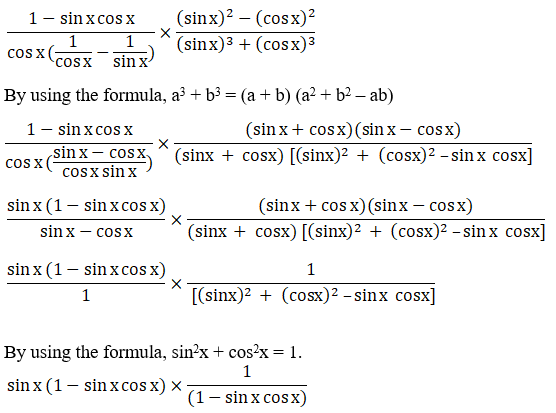sin x

= RHS

∴ LHS = RHS

Hence Proved.

6.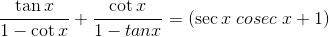Solution:

Let us consider the LHS: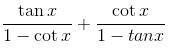By using the formula,

tan θ = sin θ / cos θ;

cot θ = cos θ / sin θ

Now,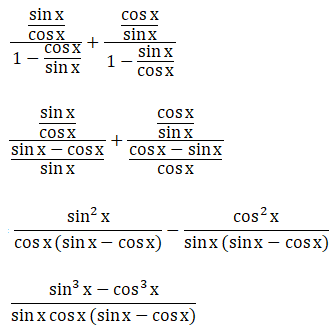By using the formula, a3 – b3 = (a – b) (a2 + b2 + ab)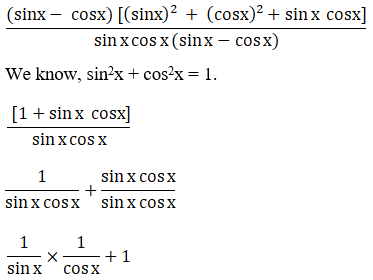By using the formula,

cosec θ = 1/sin θ,

sec θ = 1/cos θ;

cosec x × sec x + 1

sec x cosec x + 1

=RHS

∴ LHS = RHS

Hence Proved.

7.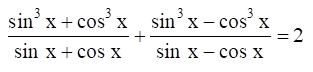Solution:

Let us consider LHS: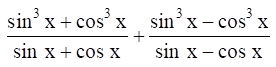By using the formula a3 ± b3 = (a ± b) (a2 + b2∓ ab)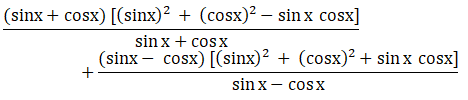We know, sin2x + cos2x = 1.

1 – sinx cosx + 1 + sinx cosx

2

= RHS

∴ LHS = RHS

Hence Proved.

8. (sec x sec y + tan x tan y)2 – (sec x tan y + tan x sec y)2 = 1

Solution:

Let us consider LHS:

(sec x sec y + tan x tan y)2 – (sec x tan y + tan x sec y)2

Expanding the above equation we get,

[(sec x sec y)2 + (tan x tan y)2 + 2 (sec x sec y) (tan x tan y)] – [(sec x tan y)2 + (tan x sec y)2 + 2 (sec x tan y) (tan x sec y)] [sec2 x sec2 y + tan2 x tan2 y + 2 (sec x sec y) (tan x tan y)] – [sec2 x tan2 y + tan2 x sec2 y + 2 (sec2 x tan2 y) (tan x sec y)]

sec2 x sec2 y – sec2 x tan2 y + tan2 x tan2 y – tan2 x sec2 y

sec2 x (sec2 y – tan2 y) + tan2 x (tan2 y – sec2 y)

sec2 x (sec2 y – tan2 y) – tan2 x (sec2 y – tan2 y)

We know, sec2 x – tan2 x = 1.

sec2 x × 1 – tan2 x × 1

sec2 x – tan2 x

1 = RHS

∴ LHS = RHS

Hence proved.

9.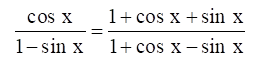Solution:

Let us Consider RHS: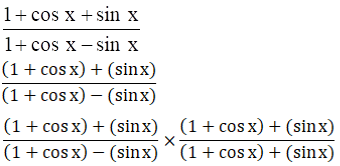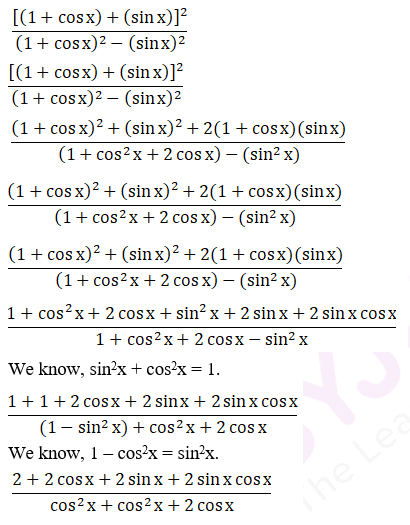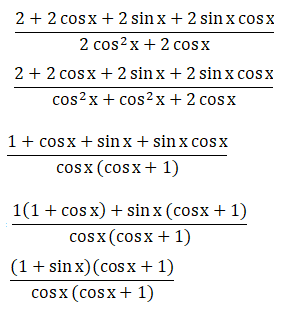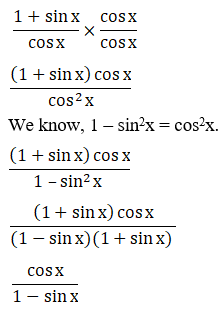= LHS

∴ LHS = RHS

Hence Proved.

10.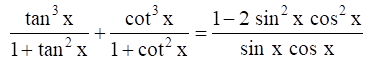Solution:

Let us consider LHS: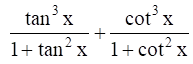By using the formulas,

1 + tan2x = sec2x and 1 + cot2x = cosec2x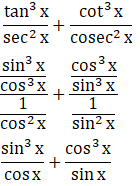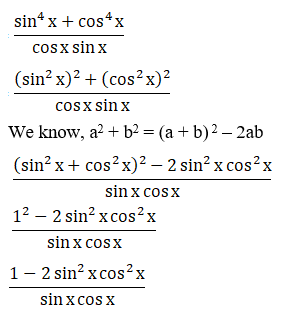= RHS

∴ LHS = RHS

Hence Proved.

11.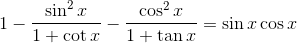Solution:

Let us consider LHS: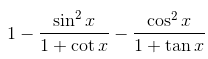By using the formula,

tan θ = sin θ / cos θ;

cot θ = cos θ / sin θ

Now,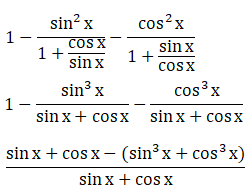By using the formula, a3 + b3 = (a + b) (a2 + b2– ab)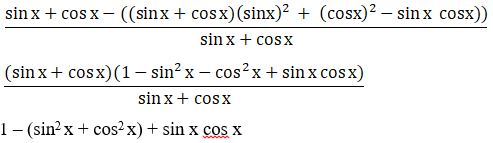We know, sin2 x + cos2 x = 1.

1 – 1 + sin x cos x

Sin x cos x

= RHS

∴ LHS = RHS

Hence proved.

12.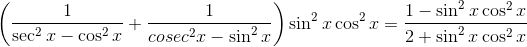Solution:

Let us consider LHS: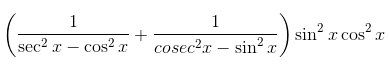By using the formula,

cosec θ = 1/sin θ,

sec θ = 1/cos θ;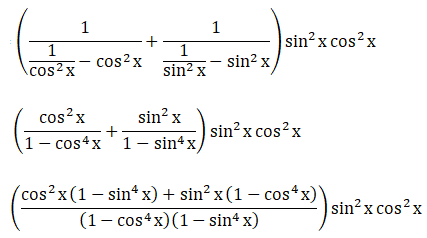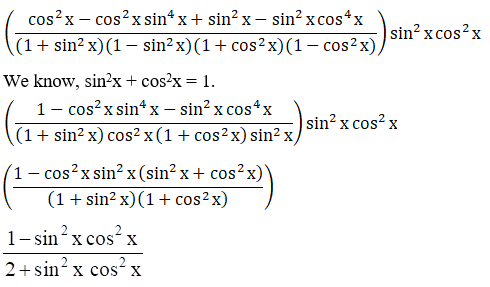= RHS

∴ LHS = RHS

Hence proved.

13. (1 + tan α tan β) 2 + (tan α – tan β) 2 = sec2 α sec2 β

Solution:

Let us consider LHS: (1 + tan α tan β) 2 + (tan α – tan β) 2

1+ tan2 α tan2 β + 2 tan α tan β + tan2 α + tan2 β – 2 tan α tan β

1 + tan2 α tan2 β + tan2 α + tan2 β

tan2 α (tan2 β + 1) + 1 (1 + tan2 β)

(1 + tan2 β) (1 + tan2 α)

We know, 1 + tan2 θ = sec2 θ

So,

sec2 α sec2 β

= RHS

∴ LHS = RHS

Hence proved.